# Radiation Biophysics Laboratory Department of Cell Biology Biophysics

• Slides: 29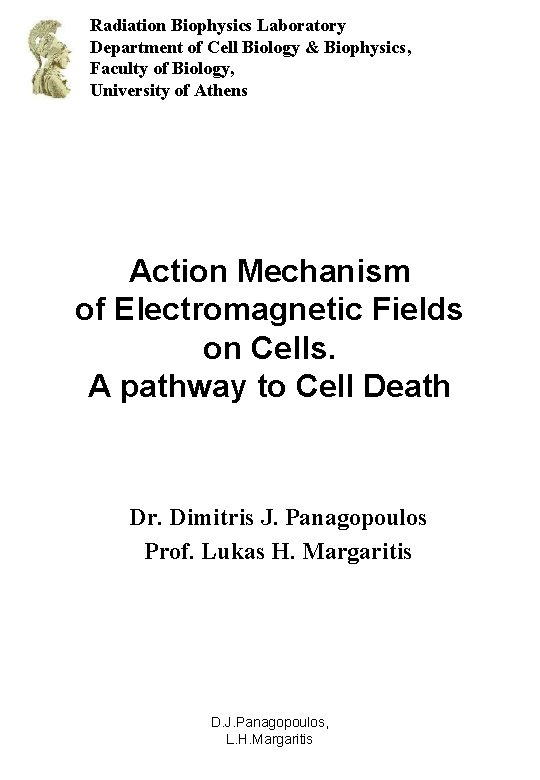Radiation Biophysics Laboratory Department of Cell Biology & Biophysics, Faculty of Biology, University of Athens Action Mechanism of Electromagnetic Fields on Cells. A pathway to Cell Death Dr. Dimitris J. Panagopoulos Prof. Lukas H. Margaritis D. J. Panagopoulos, L. H. MargaritisCell Membranes and Ion Channels Cell Membrane Ion Channel structure D. J. Panagopoulos, L. H. Margaritis 2Cation Channels Unfolded Sodium channel protein Cross section of Na+ channel with positively charged S 4 helices D. J. Panagopoulos, L. H. Margaritis 3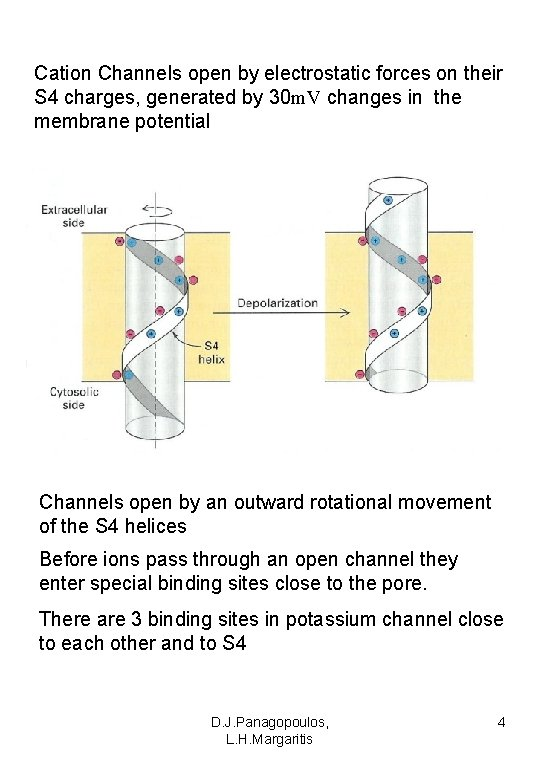Cation Channels open by electrostatic forces on their S 4 charges, generated by 30 m. V changes in the membrane potential Channels open by an outward rotational movement of the S 4 helices Before ions pass through an open channel they enter special binding sites close to the pore. There are 3 binding sites in potassium channel close to each other and to S 4 D. J. Panagopoulos, L. H. Margaritis 4Transmembrane Electrical Potential At electrochemical balance, the net movement of ions across the membrane is zero and there is a potential difference Δ between the two sides, with the internal always negative relative to the external, called Transmembrane Electrical Potential Δ = o - i 100 m. V (s 10 nm, membrane’s thickness) D. J. Panagopoulos, L. H. Margaritis 5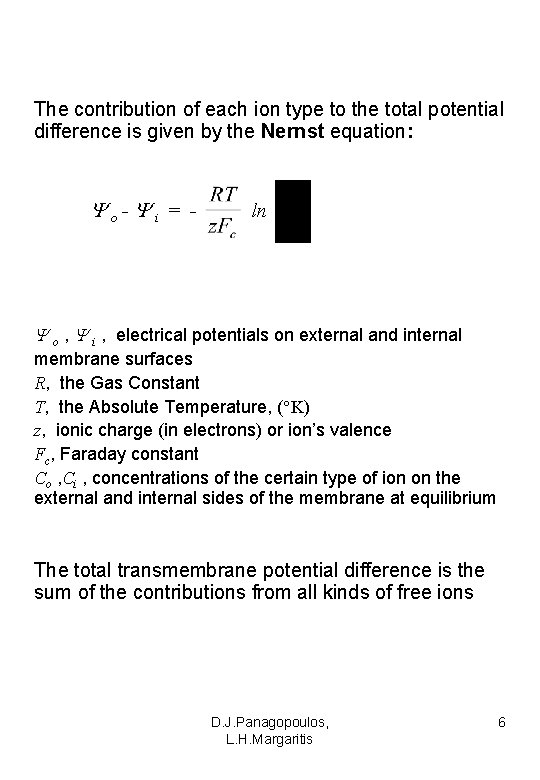The contribution of each ion type to the total potential difference is given by the Nernst equation: o- i = - ln o , i , electrical potentials on external and internal membrane surfaces R, the Gas Constant T, the Absolute Temperature, ( K) z, ionic charge (in electrons) or ion’s valence Fc, Faraday constant Co , Ci , concentrations of the certain type of ion on the external and internal sides of the membrane at equilibrium The total transmembrane potential difference is the sum of the contributions from all kinds of free ions D. J. Panagopoulos, L. H. Margaritis 6Cell’s Free Ions Transmembrane Electric Field Across all cell membranes there is a strong Electric field, ( 107 V/m), mainly determined by free ions concentration differences between the two sides of the membrane Intensity of Transmembrane Electric Field m = Δ / s 107 V/m D. J. Panagopoulos, L. H. Margaritis 7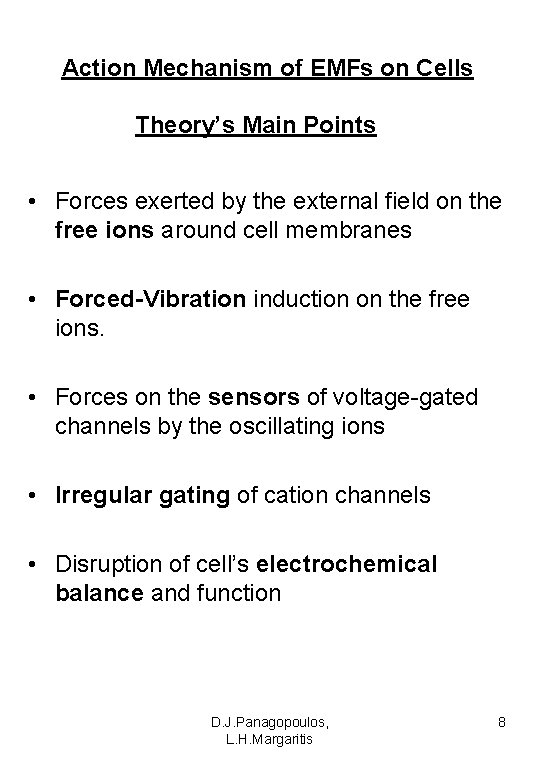Action Mechanism of EMFs on Cells Theory’s Main Points • Forces exerted by the external field on the free ions around cell membranes • Forced-Vibration induction on the free ions. • Forces on the sensors of voltage-gated channels by the oscillating ions • Irregular gating of cation channels • Disruption of cell’s electrochemical balance and function D. J. Panagopoulos, L. H. Margaritis 8Any external oscillating field exerts oscillating forces on the free ions around any cell membrane inducing a forced-vibration on the whole ionic cloud A. Electric Field Consider an External Alternating E-field = o sin t =2 D. J. Panagopoulos, L. H. Margaritis 9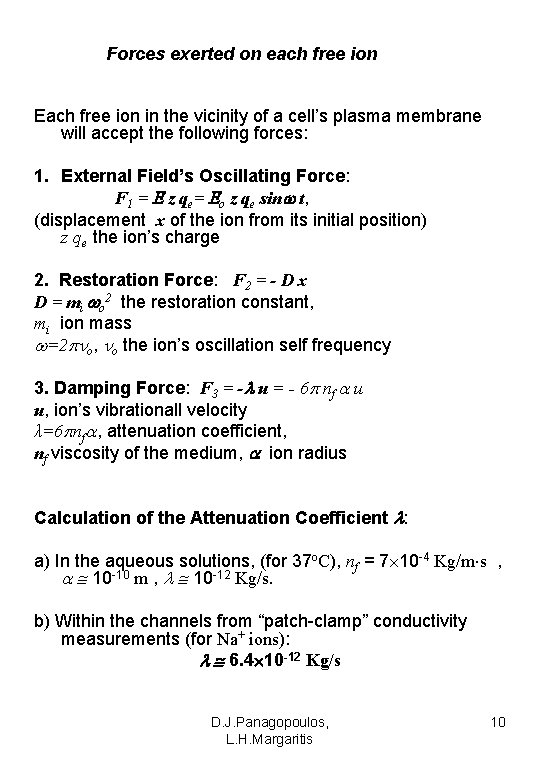Forces exerted on each free ion Each free ion in the vicinity of a cell’s plasma membrane will accept the following forces: 1. External Field’s Oscillating Force: F 1 = z qe= o z qe sin t, (displacement x of the ion from its initial position) z qe the ion’s charge 2. Restoration Force: F 2 = - D x D = mi o 2 the restoration constant, mi ion mass =2 o, o the ion’s oscillation self frequency 3. Damping Force: F 3 = - u = - 6 nf u u, ion’s vibrationall velocity λ=6 nf , attenuation coefficient, nf viscosity of the medium, ion radius Calculation of the Attenuation Coefficient : a) In the aqueous solutions, (for 37 o. C), nf = 7 10 -4 Kg/m s , 10 -10 m , 10 -12 Kg/s. b) Within the channels from “patch-clamp” conductivity measurements (for Νa+ ions): 6. 4 10 -12 Kg/s D. J. Panagopoulos, L. H. Margaritis 10Forced-Vibration Equation The ion because of the above forces will acquire acceleration a and its movement equation will be: mi a = - u - Dx + o z qe sin t mi + + mi o 2 x = o zqe sin t (1) (2 nd order linear diff. eq. with constant coefficients) • All experimental data about cytosolic free ions’ spontaneous oscillations and membrane potential spontaneous oscillations, in all types of cells show that the frequencies of these oscillations are: 0. 016 - 0. 2 Hz • We assume that the frequencies of the spontaneous ionic oscillations represent the ionic self-frequencies, o = o /2 and we adopt the value: o = 0. 1 Hz D. J. Panagopoulos, L. H. Margaritis 11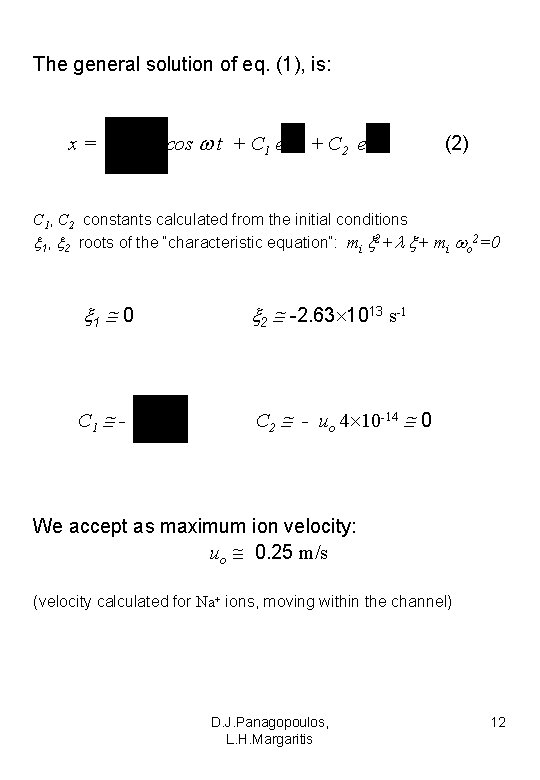The general solution of eq. (1), is: x= cos t + C 1 e + C 2 e (2) C 1, C 2 constants calculated from the initial conditions 1, 2 roots of the “characteristic equation”: mi 2+ + mi o 2=0 1 0 2 -2. 63 1013 s-1 C 1 - C 2 - uo 4 10 -14 0 We accept as maximum ion velocity: uo 0. 25 m/s (velocity calculated for Νa+ ions, moving within the channel) D. J. Panagopoulos, L. H. Margaritis 12Finally, the general solution of eq (1), is: cos t - x= (3) The constant term displaces the whole vibrational movement by: Therefore, at the moment when the external field is applied or interrupted the ion displacement is doubled pulsed EMFs are twice more bioactive than continuous EMFs. The vibrational term: x= cos t (4) Represents an harmonic oscillation of constant amplitude, independent of any initial conditions The amplitude is: A = D. J. Panagopoulos, L. H. Margaritis 13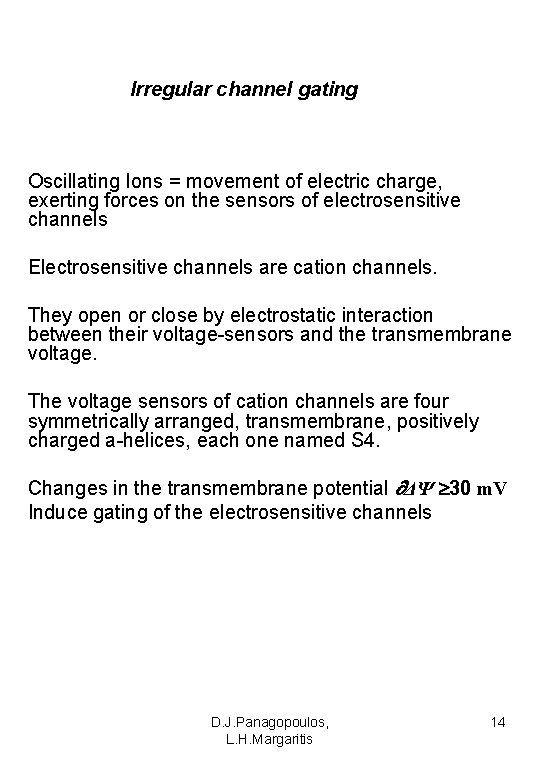Irregular channel gating Oscillating Ions = movement of electric charge, exerting forces on the sensors of electrosensitive channels Electrosensitive channels are cation channels. They open or close by electrostatic interaction between their voltage-sensors and the transmembrane voltage. The voltage sensors of cation channels are four symmetrically arranged, transmembrane, positively charged a-helices, each one named S 4. Changes in the transmembrane potential ΔΨ 30 m. V Induce gating of the electrosensitive channels D. J. Panagopoulos, L. H. Margaritis 14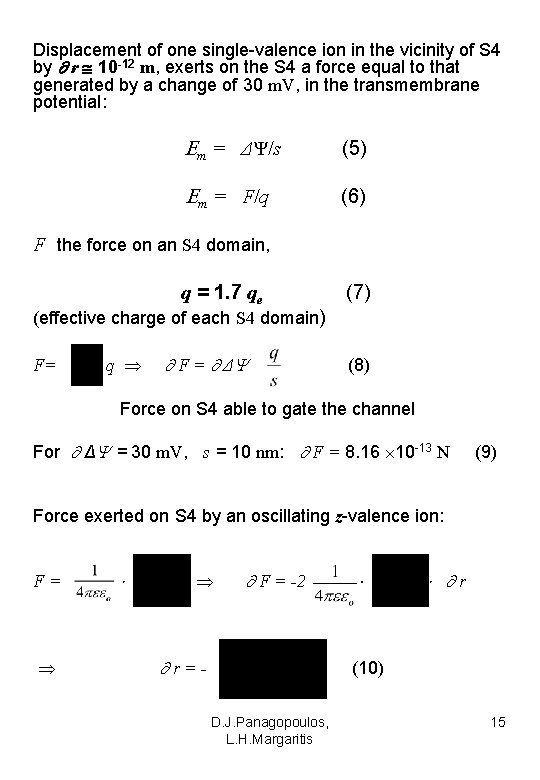Displacement of one single-valence ion in the vicinity of S 4 by r 10 -12 m, exerts on the S 4 a force equal to that generated by a change of 30 m. V, in the transmembrane potential: m = ΔΨ/s (5) m = F/q (6) F the force on an S 4 domain, q = 1. 7 qe (7) (effective charge of each S 4 domain) F= q F = Δ (8) Force on S 4 able to gate the channel For Δ = 30 m. V, s = 10 nm: F = 8. 16 10 -13 N (9) Force exerted on S 4 by an oscillating z-valence ion: F= F = -2 r=- r (10) D. J. Panagopoulos, L. H. Margaritis 15r is the necessary ion displacement in the S 4 domain, able to gate the channel We accept that the distance between the oscillating ion and the effective charge of S 4, is r 1 nm 80 (aqueous solutions) 4, (channel internal) (9), (10) r 0. 8 10 -10 m, ( = 80) r 4 10 -12 m, ( = 4). A single ion’s Displacement of 10 -12 m in the S 4 domain, can gate the channel D. J. Panagopoulos, L. H. Margaritis 16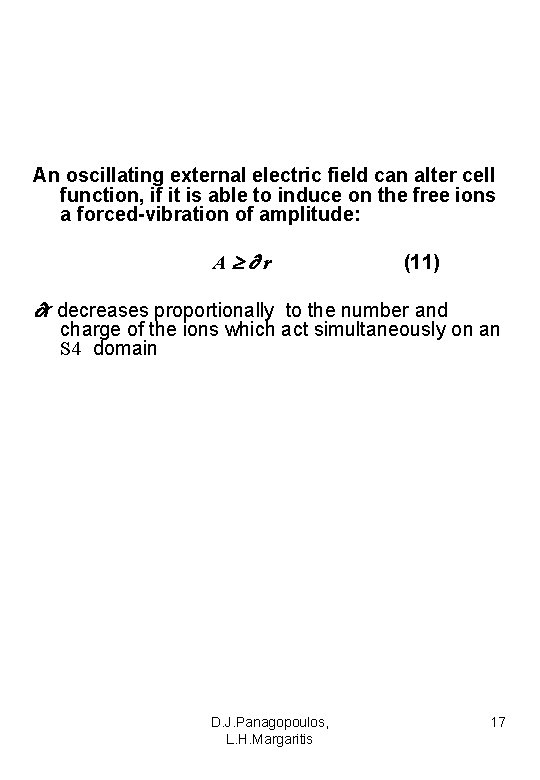An oscillating external electric field can alter cell function, if it is able to induce on the free ions a forced-vibration of amplitude: A r (11) r decreases proportionally to the number and charge of the ions which act simultaneously on an S 4 domain D. J. Panagopoulos, L. H. Margaritis 17The Thermal Motion The free ions within the same time interval are displaced at longer distances because of thermal activity than because of the forced-vibration But: Thermal motion is a random motion in every possible direction, different for every ion. It does not displace the whole ionic cloud. For u = 0. 25 m/s, the time interval is δt 1. 6 10 -11 s, for the necessary displacement r = 10 -12 m. Mean Free Path of an ion within the channel: 10 -11 m. The ion within δt, will run one mean free path in the direction of the external field and tens of free paths each one in different direction because of thermal motion. Forced-vibration is a synchronized movement of the whole ionic cloud in one direction acting additively on S 4. The tens of free paths because of thermal motion, each one in a different direction, result in mutually extinguishing opposing forces on the channel sensors, while at the same time one single displacement of the whole ionic cloud in phase results in additive forces on the sensor. D. J. Panagopoulos, L. H. Margaritis 18EMF Bioactivity Characteristics (11) 4 10 -12 m o 10 -3 log o log - 3 0 (12) (13) (Hz, V/m) (14) (one single-valence cation and continuous oscillating field) With pulsed fields, the left sides are multiplied by 2 For double-valence cations, (Ca 2+), the left sides are multiplied by 4 and the right sides divided by 2 ( r is divided by 2) bioactivity is multiplied by 8. For 2 Ca 2+ interacting simultaneously from 1 nm distance with an S 4 domain, bioactivity is multiplied by 16: 0. 25 10 -12 m o 0. 625 10 -4 log o log - 4. 2 0 (15) (16) (Hz, V/m) (17) For 3 Ca 2+ interacting simultaneously from 1 nm distance with an S 4 domain, bioactivity is multiplied by 24 D. J. Panagopoulos, L. H. Margaritis 19D. J. Panagopoulos, L. H. Margaritis 20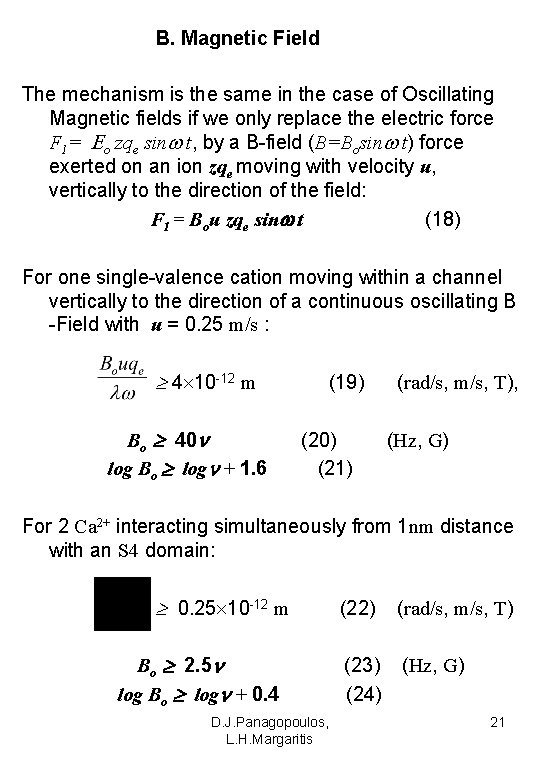B. Magnetic Field The mechanism is the same in the case of Oscillating Magnetic fields if we only replace the electric force F 1= o zqe sin t, by a B-field (B=Bosin t) force exerted on an ion zqe moving with velocity u, vertically to the direction of the field: F 1 = Bou zqe sin t (18) For one single-valence cation moving within a channel vertically to the direction of a continuous oscillating B -Field with u = 0. 25 m/s : 4 10 -12 m Bo 40 log Bo log + 1. 6 (19) (20) (21) (rad/s, m/s, T), (Hz, G) For 2 Ca 2+ interacting simultaneously from 1 nm distance with an S 4 domain: 0. 25 10 -12 m Bo 2. 5 log Bo log + 0. 4 D. J. Panagopoulos, L. H. Margaritis (22) (rad/s, m/s, T) (23) (24) (Hz, G) 21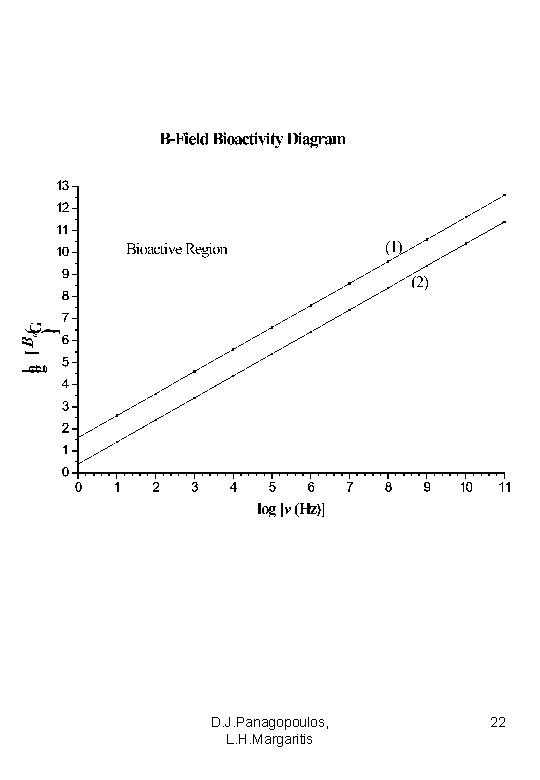D. J. Panagopoulos, L. H. Margaritis 22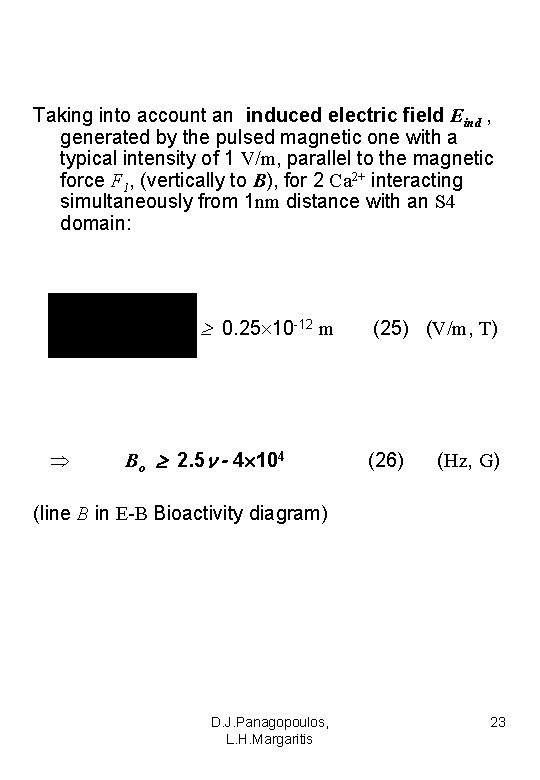Taking into account an induced electric field Eind , generated by the pulsed magnetic one with a typical intensity of 1 V/m, parallel to the magnetic force F 1, (vertically to B), for 2 Ca 2+ interacting simultaneously from 1 nm distance with an S 4 domain: 0. 25 10 -12 m Bo 2. 5 - 4 104 (25) (V/m, T) (26) (Hz, G) (line B in E-B Bioactivity diagram) D. J. Panagopoulos, L. H. Margaritis 23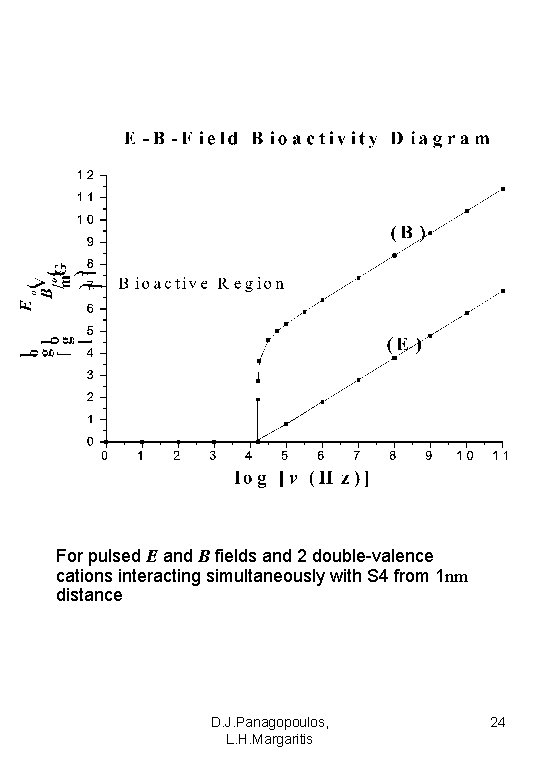For pulsed E and B fields and 2 double-valence cations interacting simultaneously with S 4 from 1 nm distance D. J. Panagopoulos, L. H. Margaritis 24For frequencies 0 -104 Hz, even very weak E-fields of less than 1 V/m, or B-fields of 1 G are able to alter cell function D. J. Panagopoulos, L. H. Margaritis 25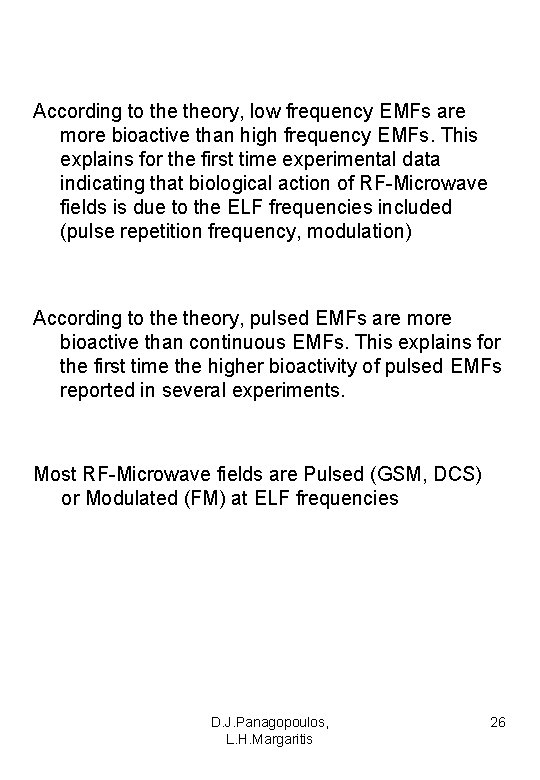According to theory, low frequency EMFs are more bioactive than high frequency EMFs. This explains for the first time experimental data indicating that biological action of RF-Microwave fields is due to the ELF frequencies included (pulse repetition frequency, modulation) According to theory, pulsed EMFs are more bioactive than continuous EMFs. This explains for the first time the higher bioactivity of pulsed EMFs reported in several experiments. Most RF-Microwave fields are Pulsed (GSM, DCS) or Modulated (FM) at ELF frequencies D. J. Panagopoulos, L. H. Margaritis 26Recent Experiments indicate DNA damage or Cell Death induction by EMFs (Panagopoulos et al. , 2007; Diem et al. , 2005; Markova et al. , 2005; Salford et al. , 2003; Lai and Singh 1995; 1996) b S 8 1 G Ovariole of a sham exposed female insect with TUNEL negative egg chambers at all the developmental stages from germarium (G) to stage 8 S 4 S 1 S 2 S 3 2 S 8 2 a Ovariole of exposed female insect with TUNEL positive signal at both check-points, germarium and stage 8 and TUNEL negative signal at the intermediate stages 2 b G 3 S 8 S 4 FC S 3 G S 7 S 2 S 1 NC OC Ovarioles of exposed female insects with TUNEL positive signals at all the developmental stages and in all types of egg chamber cells, nurse cells (NC), follicle cells (FC) and the oocyte (OC) D. J. Panagopoulos, L. H. Margaritis 27Disruption of intracellular Ionic Concentrations may lead to Cell Death 1. Irregular increase of intracellular ion concentration, may cause water molecules to enter the cell, driven by osmotic forces, proportional to the concentration increase. This can cause the cell to swell out and the plasma membrane to get ruptured, resulting to cell necrosis. 2. Perturbations of intracellular Ca+2 concentrations are responsible for apoptotic triggering. 3. A common event leading to both apoptosis and necrosis is mitochondrial membrane permeabilization, leading to increased mitochondrial concentration of Ca+2 ions D. J. Panagopoulos, L. H. Margaritis 28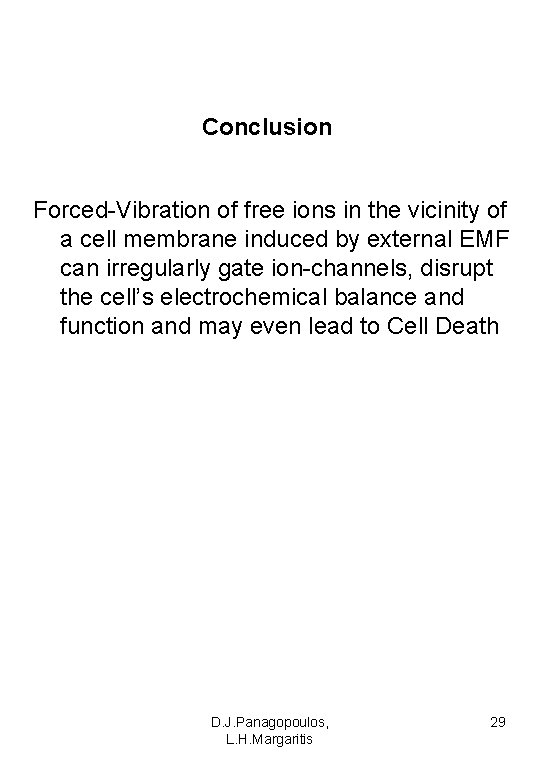Conclusion Forced-Vibration of free ions in the vicinity of a cell membrane induced by external EMF can irregularly gate ion-channels, disrupt the cell’s electrochemical balance and function and may even lead to Cell Death D. J. Panagopoulos, L. H. Margaritis 29